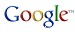VB Migration Partner

[HOWTO] Solve issues related to Null values in database fields

All VB6 database-intensive applications need to handle null values in fields. No problem exists if the original VB6 code uses the IsNull method, as in this example:

```        If IsNull(rs("BirthDate")) Then
' handle the null birthdate case here...
End If```

Unfortunately, VB6 offers many other ways to work with null fields, including appending an empty string to the field value to force the conversion into string:

```        If rs("BirthDate") & "" = "" Then
' handle both the null and empty birthdate case here...
End If```

Alas, the above statement throws a runtime exception under VB.NET or C# if the field value is Null, because the concatenation operator doesn’t work with DBNull values. The VBMigrationPartner_Support.bas module includes the FixNullValue6 method, which converts Null and Empty values to the empty string and can therefore be used to solve this issue:

```        ' this  code works well both in VB6 and VB.NET
If FixNullValue6(rs("BirthDate")) = "" Then
' handle both the null and empty birthdate case here...
End If```

If the application contains hundreds or thousands of statements such as the previous ones, you need a way to post process the result of the migration and automatically insert a call to the FixNullValue6 method. This task is quite easy if we assume that the operation of appending an empty string is meaningful only for converting Null and Empty values:

```'## project:PostProcess
"(?<val>\w+(\.Fields)?\([^)]+\)\.Value)\s+[&+]\s+(?<empty>""""|Nothing)",
"FixNullValue6(\${val})", True```

Notice that this pragma accounts for both & and + concatenation operators, and also accounts for cases when vbNullString is used instead of the “” empty string constants. When converting to C#, the pragma is slightly different:

```'## project:PostProcess
"(?<val>\w+(\.Fields)?\([^)]+\)\.Value)\s+[&+]\s+(?<empty>""""|Nothing)",
"VB6Helpers.FixNullValue(\${val})", True```

If you prefer to render these cases into VB.NET using the IsNull6 method, you can try the following set of PostProcess pragmas:

```' handle IF  rs("abc").Value & "" = "" THEN
'## project:PostProcess
"\b(?<val>\w+(\.Fields)?\([^)]+\)\.Value)\s+[&+]\s+
(?<empty>""""|Nothing)\s*=\s*\k<empty>\s+", "IsNull6(\${val})", True

' handle IF rs("abc").Value  & "" <> "" THEN
'## project:PostProcess
"\b(?<val>\w+(\.Fields)?\([^)]+\)\.Value)\s+[&+]\s+
(?<empty>""""|Nothing)\s*(>|<>)\s*\k<empty>\s+", "Not IsNull6(\${val})", True

' handle IF  Len(rs("abc").Value & "") = 0 THEN
'## project:PostProcess
"\bLen6\((?<val>\w+(\.Fields)?\([^)]+\)\.Value)\s+[&+]\s+
(?<empty>""""|Nothing)\)\s*=\s*0", "IsNull6(\${val})", True

' handle IF  Len(rs("abc").Value & "") <> 0 THEN
'## project:PostProcess
"\bLen6\((?<val>\w+(\.Fields)?\([^)]+\)\.Value)\s+[&+]\s+
(?<empty>""""|Nothing)\)\s*(>|<>)\s*0", "Not IsNull6(\${val})", True

' all previous pragmas also  handle cases when the + symbol is used instead of &,
' cases when vbNullString is used instead of "" ,
' and cases when > is  used instead of <>```

When converting to C#, the PostProcess pragmas are different:

```' handle IF  rs("abc").Value & "" = "" THEN
'## project:PostProcess
"\b(?<val>\w+(\.Fields)?\([^)]+\)\.Value)\s+[&+]\s+
(?<empty>""""|null)\s*=\s*\k<empty>\s+", "VB6Helpers.IsNull6(\${val})", True

' handle IF rs("abc").Value  & "" <> "" THEN
'## project:PostProcess
"\b(?<val>\w+(\.Fields)?\([^)]+\)\.Value)\s+[&+]\s+
(?<empty>""""|null)\s*(>|<>)\s*\k<empty>\s+", "VB6Helpers.IsNull6(\${val})", True

' handle IF  Len(rs("abc").Value & "") = 0 THEN
'## project:PostProcess
"\bVB6Helpers.Len\((?<val>\w+(\.Fields)?\([^)]+\)\.Value)\s+[&+]\s+
(?<empty>""""|null)\)\s*=\s*0", "VB6Helpers.IsNull(\${val})", True

' handle IF  Len(rs("abc").Value & "") <> 0 THEN
'## project:PostProcess
"\bVB6Helpers.Len\((?<val>\w+(\.Fields)?\([^)]+\)\.Value)\s+[&+]\s+
(?<empty>""""|null)\)\s*(>|<>)\s*0", "!VB6Helpers.IsNull(\${val})", True
```

If these pragmas don’t cover all possible cases, you can still add a pragma that inserts a call to FixNullValue6:

```' handle  IF rs("abc") & "" = rs("feg") & "" THEN
' by converting  rs("...") & "" into  FixNullValue6(rs("..."))
' IMPORTANT: this pragma must follow all the pragmas in previous list.

'## project:PostProcess "(?<val>\w+(\.Fields)?\([^)]+\)\.Value)\s+[&+]\s+(?<empty>""""|Nothing)",
"FixNullValue6(\${val})", True```
`Here is the C# version:`
```'## project:PostProcess
"(?\w+(\.Fields)?\[[^]]+\]\.Value)\s+[+]\s+(?""""|null)",
"VB6Helpers.FixNullValue(\${val})", True```

Follow Francesco Balena on VB6 migration’s group onsearch

Read Microsoft Corp’s official case study of a VB6 conversion using VB Migration Partner.

Code Architects and its partners offers remote and onsite migration services.

Subscribe to our free newsletter for useful VB6 migration tips and techniques.

A fully-working, time-limited Trial Edition of VB Migration Partner allows you to test it against your actual code

The price of VB Migration Partner depends on the size of the VB6 application, the type of license, and other factors

Migrating a VB6 application in 10 easy steps

Comparing VB Migration Partner with Upgrade Wizard

Migration tools: Feature Comparison Table